Facilitating the Spread of Knowledge and Innovation in Professional Software Development

Write for InfoQ

### Topics

InfoQ Homepage Articles Understanding and Applying Correspondence Analysis

# Understanding and Applying Correspondence Analysis

### Key Takeaways

• Simple correspondence analysis (CA) is a technique to analyze relationships between categorical variables and create profiles based on the projections of the original variables to the new dimensions that it creates
• CA begins with transformation of the raw data into a two-way contingency table that shows the frequency distribution of the variables
• Based on the proximity along the first few of the projected dimensions, we can visually explore the individuals’ and categories’ associations and can interpret a dimension generated by CA as a new, synthetic variable
• Proximity in the feature space indicates positive association, and the closer the angle between two groups/categories is to 90°, the less they are associated
• You can download a pre-packaged CA workflow from the KNIME Hub that implements data reading, preprocessing, descriptive analysis, and CA

In this article, the authors explain how correspondence analysis functions with an example of real social survey data. Also provided is an implementation of the example in KNIME Analytics Platform, an open source software, so that you can try out the analysis hands-on.

## Introduction

Customer segments, personality profiles, social classes, and age generations are examples of effective references to larger groups of people sharing similar characteristics.

The characteristics that shape these groups are often manifold and thus require multivariate analysis.

One way to access the variables is via questionnaires. Because the variables are mostly qualitative, the questionnaires produce categorical data with predefined categories, for example, on a Likert-type scale.

The starting point to analyze the relationships between categorical variables is a contingency table which compares the categories pairwise.

As the next step, correspondence analysis (CA) performs a multivariate analysis on multiple contingency tables.

It projects them into a numeric feature space, which captures most of the variability in the data by fewer dimensions.

## What Is Simple Correspondence Analysis?

Simple correspondence analysis is a technique to analyze relationships between categorical variables and create profiles based on the projections of the original variables to the new dimensions that it creates. This is useful, for example, when analyzing and visualizing survey data.

CA processes a two-way contingency table that displays the frequency distribution between two variables. It represents the frequency distribution on numeric, orthogonal dimensions. Based on the proximity along the first few of these dimensions, we can visually explore the individuals’ and categories’ associations.

We can investigate, for example, if there is a relationship between interest in politics and demographics data such as age. Also, we can interpret a dimension generated by CA as a new, synthetic dimension, such as “status,” that captures several categories which together contribute to “high” or “low” status.

## How To Perform Correspondence Analysis

### Step 1: Data collection

We start the data collection by accessing survey data, with records for N individuals who have answered K questions.

As an example, we use the European Social Survey data from the year 2018 measuring the attitudes, beliefs and behavior patterns in European nations. The data contains metadata and answers from 49,519 individuals recorded in 572 columns. We consider only a subset of the variables and perform CA to analyze the relationships between interest in politics, country, income, family relationship, gender, education, age, and internet usage.

These variables are transformed into a two-way contingency table (see the next step) based on the definition of row variables, column variables and supplemental variables as described below:

• Row variables refer to variables that represent the row IDs. In our example, the interest in politics is the row variable. It contains the following four nominal classes: not at all, hardly, quite, and very interested. The data for 98 participants who didn’t provide the information about their interest in politics (not applicable, refusal, no answer, don’t know) were discarded from the analysis.

• Column variables refer to variables that represent the column headers. The column variables are income, family relationship, gender, education, age, and internet usage.

• Supplementary variables can be used to interpret the resulting profiles, but they are not used in computing CA. In our example, “country” is the supplementary variable.

Note that if there were numeric variables, these had to be discretized before performing CA.

The survey data can be stored in varying formats, for example, in a csv file. Here, each row corresponds to an individual filling out the survey. Each column represents a survey question or metadata, such as the ID of the participant: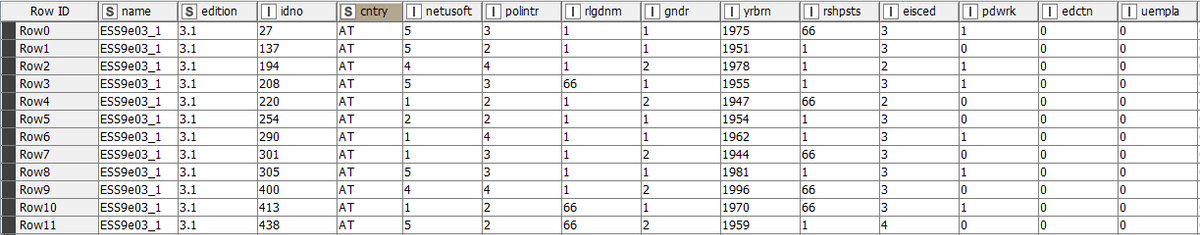Figure 1. Raw survey data as a starting point of CA

Notice that the column and row variables may need to be binned or encoded to help give a better understanding of the CA results. For example, the survey data reports 10 income deciles, which we encoded to seven income classes: very low, low, mid low, middle, mid high, high, and very high.

### Step 2: Data preprocessing

In data preprocessing, we create a two-way contingency table that shows the frequency distribution of the row and column variables.

Figure 2. below shows a part of the contingency table for the survey data in our example: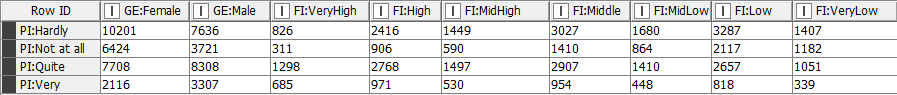Figure 2. A two-way contingency table showing the preprocessed survey data before computing CA

In the first row, it shows how the 17,837 survey participants hardly interested in politics are distributed into two categories, male and female, as well as into the seven categories describing family income. The more column variables there are, and the more categories in each column variable, the wider the table.

The transformation of the raw data into a contingency table is required to perform CA via the algorithms available, for example, in R software. In the next step, we explain how CA functions under the hood, although it is not necessary for executing such algorithms.

### Step 3: Computing CA

Projecting the data into new numeric dimensions in CA works the same way as in principal component analysis (PCA), by sequentially constructing orthogonal dimensions of the data. This can be performed by singular value decomposition.

However, while in PCA, the decomposition is based on maximizing the variance; in CA it is based on maximizing the inertia.

For each row variable i, inertia is calculated with the following formula:

Inertia (i/GJ) =fi.d2x2(i,GJ

Where fi. is the weight, i.e., the marginal sum of row variable i, and d2x2(i,GJ) is the  chi-squared distance from the mean profile defined by the marginal probabilities of column variables J. The total inertia is calculated by summing up these inertias for all row variables I. In the extreme case, if the row reflects the mean profile, the inertia of that row variable is zero.

For column variables, the inertia is the sum of inertias of their categories j:

Inertia (j/GI) = f.d2x2(j,GI),

Where f.j is the weight, the marginal sum of column variable j, and d2x2(j,GI) is the chi-squared distance from the mean profile defined by the marginal probabilities of I row variables.

The sum of inertias of all column variables j produces the same total inertia as the sum of inertias of all individuals i.

### Step 4: Interpreting the results

In this step, we explain how to interpret the results of CA visually in a scree plot and biplot and numerically via the output statistics.

Scree plot

To compare the percentages of total inertia that the new dimensions explain, we can take a look at a scree plot as shown in Figure 3: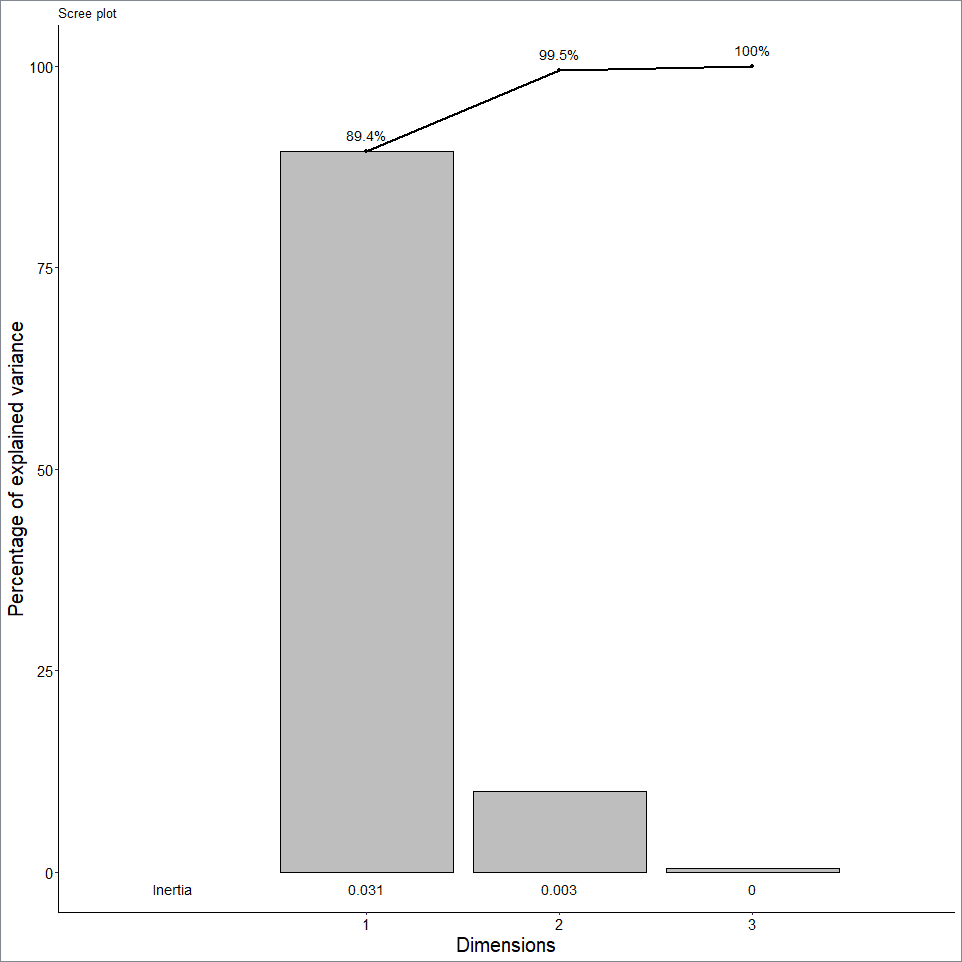Figure 3. Scree plot showing the percentages of inertia captured by the new dimensions generated by CA

In our example, the first dimension explains 89.4% of the inertia, while the second dimension explains 10.19% of it. Together, the first two dimensions explain 99.5% of the total inertia.

Biplot

Next, we project the row and column variables into the first two dimensions and explore them visually in a biplot: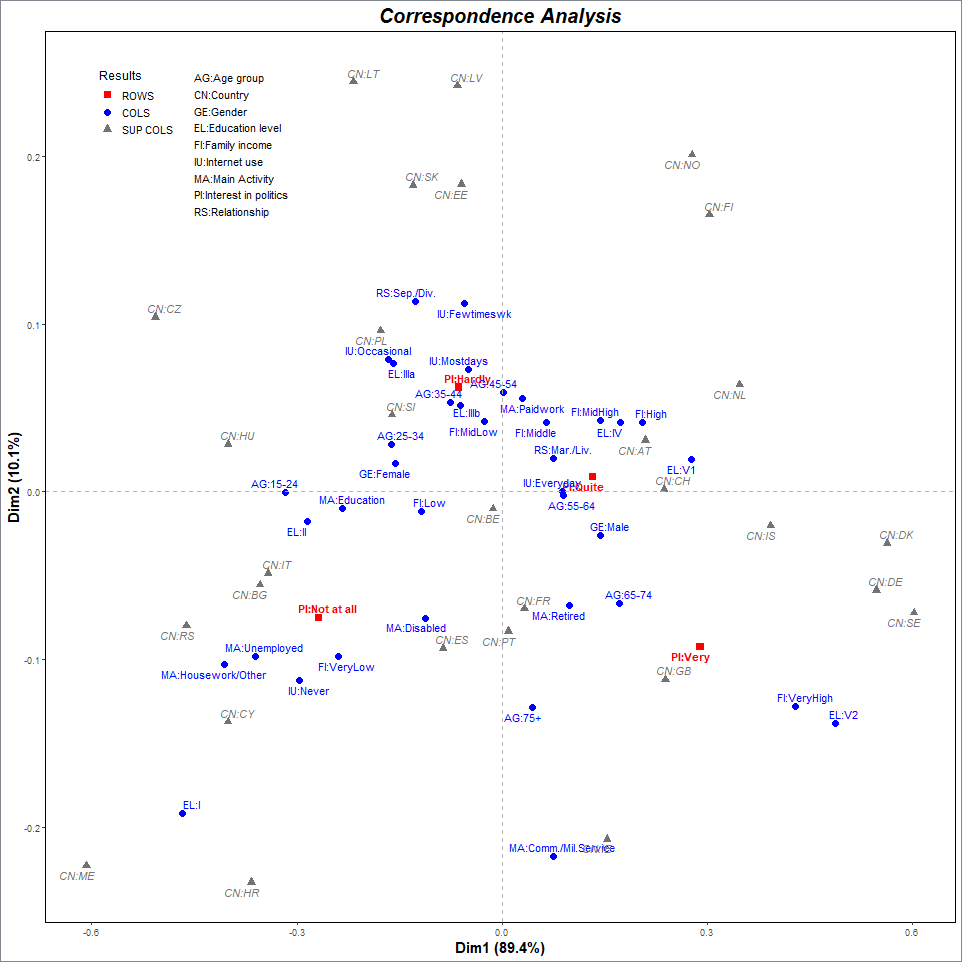Figure 4. Biplot showing the row, column and supplementary variables in two-dimensional space

The biplot shows the first two dimensions on the x- and y-axis. It is possible to show the row, column and supplementary variables along the same axes using transition formulas between the coordinates of row, column and supplementary variables.

Proximity in the feature space indicates positive association. For example, the group of individuals who are very interested in politics (PI: Very) is close to the category of very high income (FI:VeryHigh), and these variables are therefore strongly associated. Also, the categories of very high income and MA level education (EL: V2) are strongly associated. This implies that people with very high income have MA level education more often than an average person from any income class.

Also, the closer the angle between two groups/categories is to 90°, the less they are associated. For example, the categories “IU: Everyday” and “Age: 45-54” lie on the x- and y-axis, respectively. Therefore, this association is very weak. The contingency table in Figure 5 below confirms this: There is little deviation between the observed and expected value.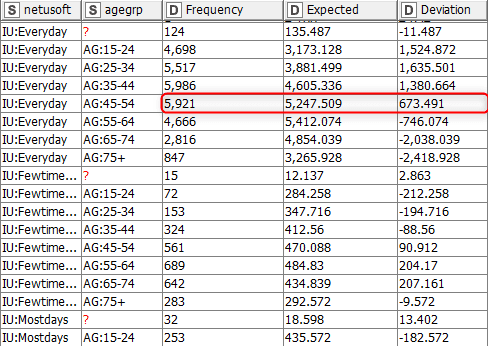Figure 5. A sample of a contingency table between analyzed variables

The categories of the supplementary variable, i.e., the countries, help to interpret the dimensions. It seems that Switzerland (CN:CH) and the Nordic countries such as Denmark and Sweden (CN:DK and CN:SE) are strongly associated with the first dimension. Instead, the Baltic countries such as Estonia and Latvia (CN:EE and CN:LV) are strongly associated with the second dimension.

## Statistics

Finally, we can inspect the groups, categories and new dimensions by looking at the output statistics. The table in Figure 6 shows a sample of the output statistics of CA for our example: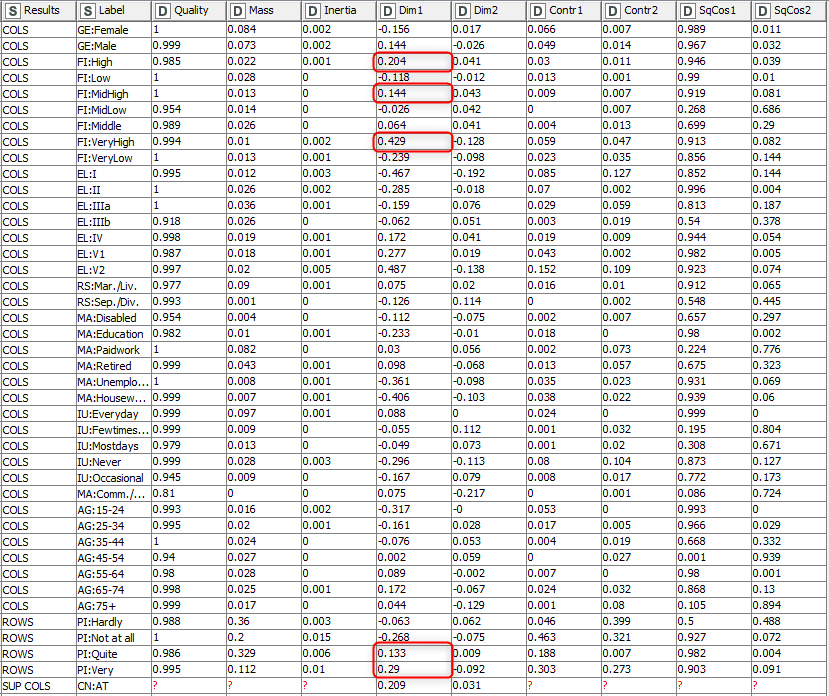Figure 6 . The output statistics of CA

The table contains the variables as row IDs and the statistics as column headers. For supplementary columns, there are no statistics, except dimensions 1 and 2, because they don’t contribute to the dimensions. Dimension 1 seems to relate positively to high levels of interest in politics, family income, education, age group, etc. Therefore, Dimension 1, which is highly important, could be interpreted as a “status” dimension (high vs. low).

In the table below, we explain what the output statistics of CA quantify and state an example question that we can answer by them.

 Statistics Definition Question Mass The relative frequency of each variable. The percentages of row and column variables, respectively, sum up to 1. Which group of individuals/ category is the most/least frequent? Inertia The inertia of a single row/column variable How diverse is each group of individuals/category? Dim1-2 The coordinates of the variable in the reduced feature space as defined by dimensions 1 and 2 How to visualize the variables in a two-dimensional feature space? Contr1-2 The percentage of the variable’s inertia of the total inertia of dimension 1 and 2, respectively Which variable explains the most variation of dimensions 1 and 2? SqCos1-2 The percentage of the variable’s inertia projected onto dimension 1 and 2, respectively How well do dimension 1 and 2 alone explain the variation in this variable? Quality The representation quality of the variable by dimensions 1 and 2 together. Equal to the sum of SqCos 1–2. Value 1 corresponds to a perfect representation. How well do dimensions 1 and 2 together explain the variation in this variable?

Table 1. Definitions and interpretations of the output statistics produced by CA

Next, we show how to perform CA and produce the results introduced above in KNIME Analytics Platform.

## Practical Implementation of CA

In this section, we will introduce how to perform the example application of this article, analyzing social survey data via CA, in KNIME Analytics Platform. The KNIME workflow below shows the steps.

You can download the Exploring categorical data via Correspondence Analysis workflow from the KNIME Hub and open it in KNIME Analytics Platform. KNIME Analytics Platform is open source and can be downloaded from the KNIME website.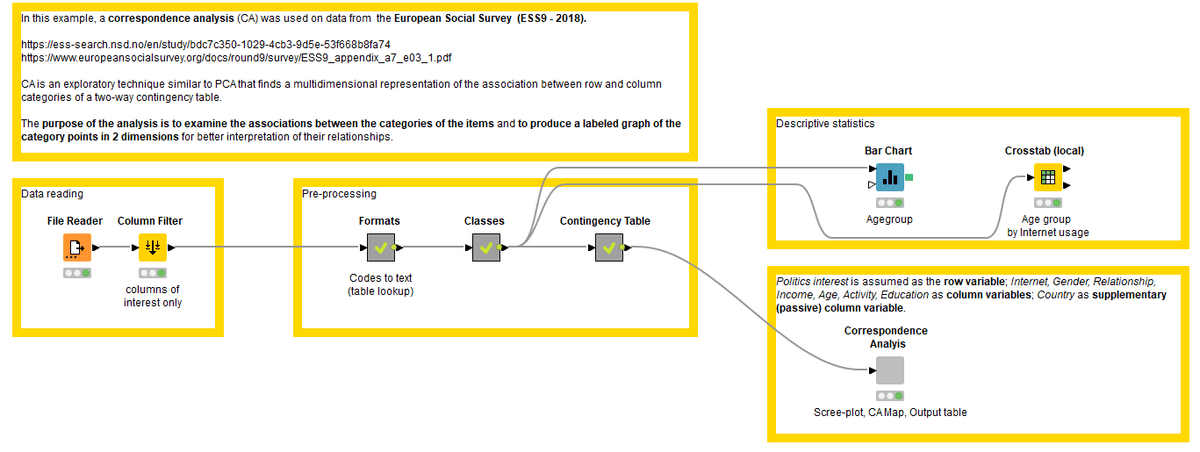Figure 7. Example of KNIME workflow performing CA on European Social Survey data. You can download the workflow from the KNIME Hub.

The workflow progresses in four steps: data reading, preprocessing, descriptive analysis, and CA.

First, it accesses the data as a CSV file, which stores the data as shown in Figure 1.

Second, it accesses the dictionaries that contain the descriptions of the codes to replace them in the data. After that, it bins some of the variables into fewer categories. Then, it creates the contingency table as shown in Figure 2.

Lastly, it computes the CA and produces a view that displays the scree plot, biplot, and statistics table (Figures 3-4 and Table 1). It performs CA using functions of the R software, in particular, the ca () function of the ca package. For the views, it uses the function ggplot () of the ggplot2 package. The KNIME Interactive R Statistics Integration allows us to write the script within the visual workflow.

In addition, it displays a bar chart and contingency table to explore the frequency distributions in the data in parallel to performing CA (Figure 5).

## Summary

In this article, we introduced correspondence analysis, which analyzes associations in categorical data, and showed how it helps to analyze categorical data beyond a contingency table by projecting the categories of the variables onto new numeric dimensions. You can find these associations based on the proximity of the variables in a reduced feature space that could not otherwise be discovered through a pairwise analysis.

Style

## Hello stranger!

You need to Register an InfoQ account or or login to post comments. But there's so much more behind being registered.

Get the most out of the InfoQ experience.

Allowed html: a,b,br,blockquote,i,li,pre,u,ul,p